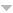Name: Higor Henrique Aranda Cotta
Type: PhD thesis
Publication date: 22/08/2019

Examining board:

Name RoleNeyval Costa Reis Jr. Internal Examiner *
Taciana Toledo de Almeida Albuquerque Internal Examiner *
Wilfredo Omar Palma Manriquez External Examiner *
Alexandre Renaux External Examiner *
Glaura da Conceição Franco External Examiner *
Márton Ispány External Examiner *

Summary: This manuscript proposes new robust estimation methods for the autocovariance and autocorrelation matrices functions of stationary multivariates time series that may have random additives outliers. These
functions play an important role in the identification
and estimation of time series model parameters. Random additive outliers can impact the level of one or
more components of the multivariate vector. This increases the overall variability of the series, which has
an impact on the periodogram matrix and leads to
a decrease in the values of the autocorrelation matrix function. We first propose new estimators of the
autocovariance and of autocorrelation matrices functions constructed using a spectral approach considering the periodogram matrix periodogram which is the
natural estimator of the spectral density matrix. As in
the case of the classic autocovariance and autocorrelation matrices functions estimators, these estimators are affected by aberrant observations. Thus, any
identification or estimation procedure using them is directly affected, which leads to erroneous conclusions.
To mitigate this problem, we propose the use of robust
statistical techniques to create estimators resistant to
aberrant random observations.
As a first step, we propose new estimators of autocovariance and autocorrelation functions of univariate
time series. The time and frequency domains are linked by the relationship between the autocovariance
function and the spectral density. As the periodogram
is sensitive to aberrant data, we get a robust estimator by replacing it with the M-periodogram. The
M-periodogram is obtained by replacing the Fourier
coefficients related to periodogram calculated by the
standard least squares regression with the ones calculated by the M-robust regression. The asymptotic
properties of estimators are established. Their performances are studied by means of numerical simulations for different sample sizes and different scenarios of contamination. The empirical results indicate
that the proposed methods provide close values of
those obtained by the classical autocorrelation function when the data is not contaminated and it is resistant to different contamination scenarios. Thus, the
estimators proposed in this thesis are alternative methods that can be used for time series with or without
outliers.
The estimators obtained for univariate time series are
then extended to the case of multivariate series. This
extension is simplified by the fact that the calculation
of the cross-periodogram only involves the Fourier coefficients of each component from the univariate series. Again, the duality relationship between time and
frequency domains is considered via the link between
the autocovariance matrix function and the spectral
density matrix stationary multivariate time series. The
M-periodogram matrix is a robust periodogram matrix
alternative to build robust estimators of the autocovariance and autocorrelation matrices functions. The
asymptotic properties are studied and numerical experiments are performed. As an example of an application with real data, we use the proposed functions
to adjust an autoregressive model by the Yule-Walker
method to Pollution data collected in the Vitoria re- ´
gion Brazil (particles smaller than 10 micrometers in
diameter, PM10).
Finally, the robust estimation of the number of factors in large factorial models is considered in order
to reduce the dimensionality. It is well known that the
values random additive outliers affect the covariance
and correlation matrices and the techniques that depend on the calculation of their eigenvalues and eigenvectors, such as the analysis principal components and the factor analysis, are affected. Thus, in
the presence of outliers, the information criteria proposed by Bai & Ng (2002) tend to overestimate the
number of factors. To alleviate this problem, we proposeto replace the standard covariance matrix with
the robust covariance matrix proposed in this manuscript. Our Monte Carlo simulations show that, in the
absence of contamination, the standard and robust
methods are equivalent. In the presence of outliers,
the number of estimated factors increases with the
non-robust methods while it remains the same using
robust methods. As an application with real data, we
study pollutant concentrations PM10 measured in the
Ile-de-France region of France.